Courses

# Facts That Matter, Ex 12.1 NCERT Solutions- Areas Related to Circles Class 10 Notes | EduRev

## Class 10 : Facts That Matter, Ex 12.1 NCERT Solutions- Areas Related to Circles Class 10 Notes | EduRev

The document Facts That Matter, Ex 12.1 NCERT Solutions- Areas Related to Circles Class 10 Notes | EduRev is a part of the Class 10 Course Mathematics (Maths) Class 10.
All you need of Class 10 at this link: Class 10

Facts that Matter
➢ Perimeter and Area of a Circle

• The total distance (perimeter) around a circle is called its circumference.
• The plane surface enclosed in a circle is called its area.
• If ‘r’ be the radius of a circle, then
(i) Circumference of the circle = 2πr
(ii) Area of the circle = πr2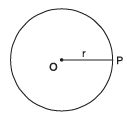Note:
(i) The interior of a circle along with its boundary is called the circular region of the circle.
(ii)
By the area of a circle, we mean the area of the circular region.

➢ Area of Sector and Segment of a Circle

• The portion (or part) of the circular region enclosed by two radii and the corresponding arc is called a sector of the circle.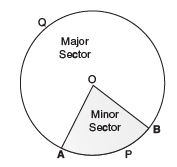• The portion (or part) of the circular region enclosed between a chord and the corresponding arc is called a segment of the circle.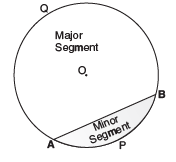Note:
(i) ∠AOB is called the ‘angle of sector’.
(ii)
OAPB is the ‘minor sector’ and OAQB is the ‘major sector’.
(iii)
APB is the ‘minor segment’ and AQB is the ‘major segment’.
(iv)
When we write ‘sector’ and ‘segment’ we will mean the ‘minor-sector’ and the ‘minor-segment’ respectively.

➢ Let us remember that

• Area of the sector of ‘angle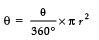• Length of the arc of a sector of angle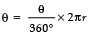• Area of a segment = [Area of the corresponding sector] − [Area of the corresponding triangle]

Page No. 225

Exercise 12.1

[Unless stated otherwise, use π = 22/7]
Q.1. The radii of the two circles are 19 cm and 9 cm respectively. Find the radius of the circle which has circumference equal to the sum of the circumferences of the two circles.
Sol. We have, r1 = 19 cm
r2 = 9 cm
Circumference of circle-I = 2πr1 = 2π (19) cm
Circumference of circle-II = 2πr2 = 2π (9) cm
Sum of the circumferences of circle-I and circle-II = 2π (19) + 2π (9)
= 2π (19 + 9) cm = 2π (28) cm
Let R be the radius of the circle-III.
∴ Circumference of circle-III = 2πR
According to the condition:
2πR = 2π (28)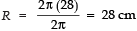Thus, the radius of the new circle = 28 cm

Q.2. The radii of two circles are 8 cm and 6 cm respectively. Find the radius of the circle having area equal to the sum of the areas of the two circles.
Sol. We have, Radius of circle-I, r1 = 8 cm
Radius of circle-II, r2 = 6 cm
Area of circle-I = πr12 = π (8)2 cm2
Area of circle-II = πr22 = π (6)2 cm2
Let the area of the circle-III be R
∴ Area of circle-III = πR2
Now, according to the condition:

π r12 + π r22 = πR2
i.e. π (8)2 + π (6)2 = πR2
⇒ π (82 + 62)= πR2
⇒ 82 + 62 = R2
⇒ 64 + 36 = R2
⇒ 100 = R2
⇒ 102 = R2 ⇒ R = 10
Thus, the radius of the new circle = 10 cm.

Q.3. Figure depicts an archery target marked with its five scoring regions from the centre outwards as Gold, Red, Blue, Black and White. The diameter of the region representing gold score is 21 cm and each of the other bands is 10.5 cm wide. Find the area of each of the five scoring regions.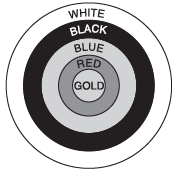Sol. Diameter of the innermost region = 21 cm
Radius of the innermost (Gold Scoring) region = 21/2 = 10.5 cm
► Area of Gold region = π (10.5)2 cm2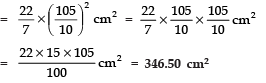Area of the Red region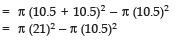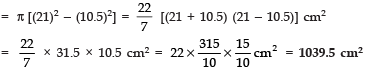Area of Blue region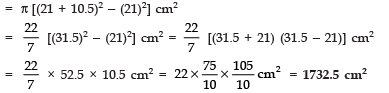Area of Black region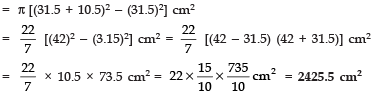Area of White region
= π [(42 + 10.5)2] = (42)2 cm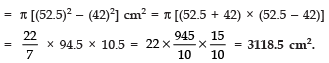Page No. 226

Q.4. The wheels of a car are of diameter 80 cm each. How many complete revolutions does each wheel make in 10 minutes when the car is travelling at a speed of 66 km per hour?
Sol. The diameter of a wheel = 80 cm
Radius of the wheel = 80/2 = 40 cm
Circumference of the wheel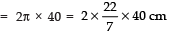⇒ Distance covered by a wheel in one revolution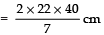Distance travelled by the car in 1hr = 66 km = 66 × 1000 × 100 cm
∴ Distance travelled in 10 minutes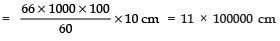Now,
Number of revolutions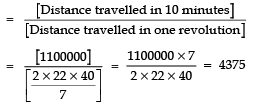Thus, the required number of revolutions = 4375

Q.5. Tick the correct answer in the following and justify your choice: If the perimeter and the area of a circle are numerically equal, then the radius of the circle is
(a) 2 units
(b) π units
(c) 4 units
(d) 7 units
Ans. (a)
Sol. We have
[Numerical area of the circle] = [Numerical circumference of the circle]
⇒ π r2 = 2πr
⇒π r2 − 2πr = 0
⇒ r2 − 2r = 0
⇒ r (r − 2) = 0r = 0 or r = 2
But r cannot be zero
∴ r = 2 units.
Thus, option (a) is correct.

Offer running on EduRev: Apply code STAYHOME200 to get INR 200 off on our premium plan EduRev Infinity!

## Mathematics (Maths) Class 10

62 videos|363 docs|103 tests

,

,

,

,

,

,

,

,

,

,

,

,

,

,

,

,

,

,

,

,

,

,

,

,

;